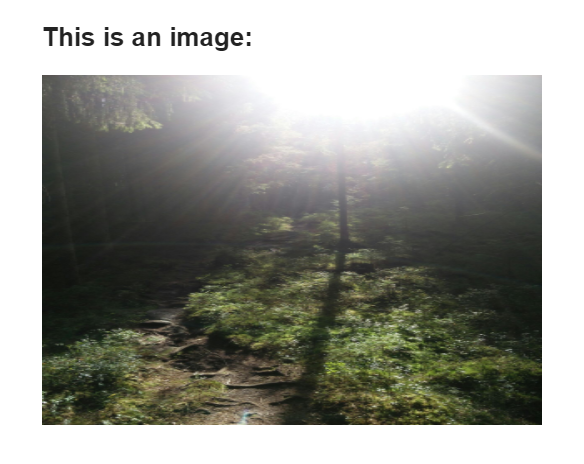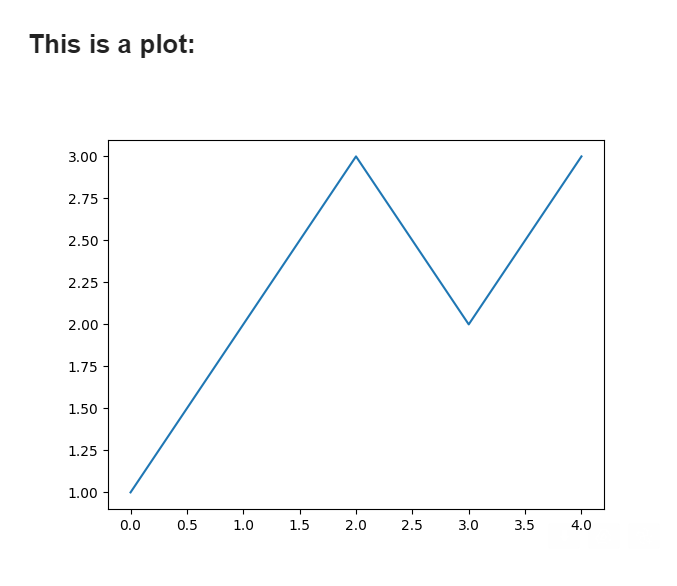# Sending Email with Image in Body¶

With Red Mail you can also embed an image directly to the HTML body of an email to make them more visual.

Red Mail supports various types for the image:

## Embedding Image from path¶

You can also embed images straight to the HTML body of the email:

```email.send(
subject='An image',
html="""
<h1>This is an image:</h1>
{{ my_image }}
""",
body_images={
'my_image': 'path/to/image.png',
}
)
```

The outcome looks like this:The image will be rendered as `<img src="cid:...">`. In case you need to control the image (like the size) you can also create the `img` tag yourself:

```email.send(
subject='An image',
html="""
<h1>This is an image:</h1>
<img src="{{ my_image.src }}" width=500 height=350>
""",
body_images={
'my_image': 'path/to/image.png',
}
)
```

In addition to paths as strings, the following are supported:

## Embedding Image from bytes¶

You may also pass the image as bytes:

```import base64

# data of a simple PNG image
data = 'iVBORw0KGgoAAAANSUhEUgAAAAUAAAAFCAYAAACNbyblAAAAHElEQVQI12P4//8/w38GIAXDIBKE0DHxgljNBAAO9TXL0Y4OHwAAAABJRU5ErkJggg=='
data_as_bytes = base64.b64decode(data_as_base64)

gmail.send(
subject='An image',
html="""
<h1>This is an image:</h1>
{{ myimage }}
""",
body_images={
'myimage': data_as_bytes
},
)
```

Note

The bytes are expected to represent a PNG image. In case your image is in other format (ie. JPEG), you should specify the image using the dict format.

## Embedding Image with dict format¶

You may also include images using the dict format:

```import base64

# data of a simple PNG image
data = 'iVBORw0KGgoAAAANSUhEUgAAAAUAAAAFCAYAAACNbyblAAAAHElEQVQI12P4//8/w38GIAXDIBKE0DHxgljNBAAO9TXL0Y4OHwAAAABJRU5ErkJggg=='
data_as_bytes = base64.b64decode(data_as_base64)

gmail.send(
subject='An image',
html="""
<h1>This is an image:</h1>
{{ myimage }}
""",
body_images={
'myimage': {
'myimage': data_as_bytes,
'subtype': 'png'
}
}
)
```

Compared to embedding bytes, using the dict format you can also specify the `subtype` of the image.

## Embedding Figure¶

As mentioned, you may also include Matplotlib figures directly to the email. This is especially handy if you are creating automatic statistics.

A simple example to include a figure:

```# Create a simple plot
import matplotlib.pyplot as plt
fig = plt.figure()
plt.plot([1,2,3,2,3])

# Send the plot
email.send(
subject='A plot',
html="""
<h1>This is a plot:</h1>
{{ my_plot }}
""",
body_images={
'my_plot': fig,
}
)
```

The outcome looks like this:## Embedding Pillow Image¶

You may also include Pillow image:

```# Create a simple image
from PIL.Image import Image
img = Image.new('RGB', (100, 30), color = (73, 109, 137))

# Send the plot
email.send(
subject='A PIL image',